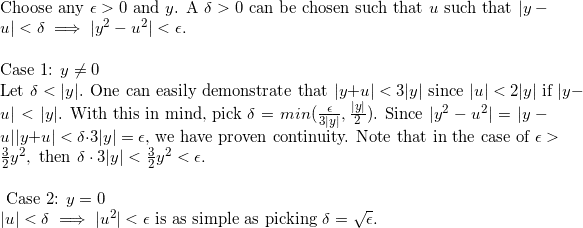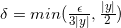# Continuity of y^2

I wrote up a proof for the continuity of y^2 for practice. Is this acceptable in the context of a Real Analysis I course?QED

Thanks!

Office_Shredder
Staff Emeritus
Gold Member
2021 Award
Case one is only taken care of when epsilon is big, which is the uninteresting case. The fact that delta is not a function of epsilon should be an immediate giveaway that you haven't constructed a proof

Case one is only taken care of when epsilon is big, which is the uninteresting case. The fact that delta is not a function of epsilon should be an immediate giveaway that you haven't constructed a proof

I think I do address this. I set ##\delta = \frac{\epsilon}{3|y|}## when ##\frac{\epsilon}{3|y|} < \frac{|y|}{2}## so that the restriction ##\delta < |y|## is maintained. In the proof I state.

Office_Shredder
Staff Emeritus
Gold Member
2021 Award
That's what I get for trying to read the forums on my cell phone at one in the morning. OK, I re-read it on my laptop and I think it looks pretty good.... your original statement doesn't actually say what u is (it looks like it gets cut off) and I think any correct statement should specify that delta depends on u - in particular you should say for any u we can show there exists delta, as opposed to there exists delta for any u.

When you write
$$\delta 3 |y| = \epsilon$$
that should really be a less than or equal to sign, because if delta happened to be |y|/2 (in the event where epsilon IS big) then this is not going to be an equality. Also the proof would be better structured by removing the "One can easily see that.... with this in mind pick delta " and replace it with writing down what delta is going to be equal to, and then stating all the consequences - your proof does not require you to explain why you make a choice of definition before stating it (this is something that took me a long time to get over doing and I still do from time to time, and it is a bad habit). It's not wrong but it's not the cleanest way to structure a final proof.

•1 person
Thanks Office_Shreader for your feedback! I will try to amend my proof accordingly:

Let ##f:\mathbb{R} \to \mathbb{R}## be a function such that ## y \mapsto y^2##. To show continuity at every point of ##f##, it is sufficient to demonstrate:
##\forall u \;\; \forall \epsilon > 0 \;\; \exists \delta : \forall y \; \left ( |y-u| < \delta \implies |y^2 - u^2| \right ) ##​

Choose any ##\epsilon > 0## and real ##u##.

Case 1: ##u=0##
Let ##\delta = \sqrt{\epsilon}##. It is clear that ##|y| < \sqrt{\epsilon} \implies |y^2| = \epsilon##.​

Case 2: ##u \neq 0##
Choose any ##\delta > 0## such that ##\delta \leq \frac{\epsilon}{3|u|}## and ##\delta \leq \frac{|u|}{2}##. (Note: If ##|y-u| \geq \delta## then the continuity condition is met and the argument is complete; assume ##|y-u| < \delta## holds for the remainder of the proof) Since ##\delta \leq \frac{|u|}{2} < |u|##, it follows ##|y+u| < 3|u|##. Therefore, ##|y^2-u^2| = |y+u||y-u| < 3|u| \delta \leq \epsilon ## ■

I want to make sure of a few things:
1. ##\delta## can be a function of both ##\epsilon## and ##u## (but not ##y##) for pointwise continuity since they both proceed ##\delta##'s quantifier?
2. Is ##(|y-u| < \delta) \wedge (\delta < |u|) \implies |y+u| < 3|u|## obvious enough to state without proof?
3. Obviously, once ##\delta## is chosen, ##|y-u|## could be ##\geq \delta## since both were chosen arbitrarily and without respect to ##\delta##. If this inequality does not hold, neither do the following arguments. Is the parenthetical "(Note: If ##|y-u| \geq \delta## then the continuity condition is met and the argument is complete; assume ##|y-u| < \delta## holds for the remainder of the proof)" sufficient to tackle this problem?

mathwonk
Homework Helper
to me the easiest way to see this is to write y as y0+d. then (y0+d)^2 = y0^2 + 2dy0 + d^2.

then to have y^2 - y0^2 < e, you just have to make both 2dy0 and d^2 less than e/2.

e.g. take d < min(1, e/2), and also d < e/(2y0).

oh now i see why you took y0 ≠ 0. but of course if y0 = 0, then 2dy0 is always < e/2 for any d, so you don't need to worry about that case.

Office_Shredder
Staff Emeritus
Gold Member
2021 Award
Let ##f:\mathbb{R} \to \mathbb{R}## be a function such that ## y \mapsto y^2##. To show continuity at every point of ##f##, it is sufficient to demonstrate:
##\forall u \;\; \forall \epsilon > 0 \;\; \exists \delta : \forall y \; \left ( |y-u| < \delta \implies |y^2 - u^2| \right ) ##​
You need a < ε at the end here, but the statement looks good other than that.

Case 1: ##u=0##
Let ##\delta = \sqrt{\epsilon}##. It is clear that ##|y| < \sqrt{\epsilon} \implies |y^2| = \epsilon##.​

It should read $|y^2| < \epsilon$

1. ##\delta## can be a function of both ##\epsilon## and ##u## (but not ##y##) for pointwise continuity since they both proceed ##\delta##'s quantifier?

1. Yes, this is correct.

[*]Is ##(|y-u| < \delta) \wedge (\delta < |u|) \implies |y+u| < 3|u|## obvious enough to state without proof?

I would probably include the proof for this but I don't think one way or the other matters that much.

[*] Obviously, once ##\delta## is chosen, ##|y-u|## could be ##\geq \delta## since both were chosen arbitrarily and without respect to ##\delta##. If this inequality does not hold, neither do the following arguments. Is the parenthetical "(Note: If ##|y-u| \geq \delta## then the continuity condition is met and the argument is complete; assume ##|y-u| < \delta## holds for the remainder of the proof)" sufficient to tackle this problem?

Anybody who's reading this proof is going to know that you only care about when |y-u|<δ, so you're OK with just noting it. You should be OK just saying "Pick any δ>0 such that...... and assume that |y-u| < δ" instead of writing a whole three lines about it.